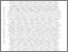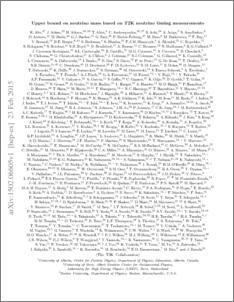Upper bound on neutrino mass based on T2K neutrino timing measurements

Nowak, Jaroslaw Andrzej and Kormos, Laura Lee and O'Keeffe, Helen Mary and Finch, Alexander John and Ratoff, Peter Neil and Dealtry, Thomas James (2016) Upper bound on neutrino mass based on T2K neutrino timing measurements. Physical Review D, 93 (1). ISSN 1550-7998Preview
PDF (1502.06605v1)
1502.06605v1.pdf - Accepted Version

The Tokai to Kamioka (T2K) long-baseline neutrino experiment consists of a muon neutrino beam, produced at the J-PARC accelerator, a near detector complex and a large 295km distant far detector. The present work utilizes the T2K event timing measurements at the near and far detectors to study neutrino time of flight as function of derived neutrino energy. Under the assumption of a relativistic relation between energy and time of flight, constraints on the neutrino rest mass can be derived. The sub-GeV neutrino beam in conjunction with timing precision of order tens of ns provide sensitivity to neutrino mass in the few MeV/$c^2$ range. We study the distribution of relative arrival times of muon and electron neutrino candidate events at the T2K far detector as a function of neutrino energy. The 90% C.L. upper limit on the mixture of neutrino mass eigenstates represented in the data sample is found to be m\$_{\nu}^2View Item